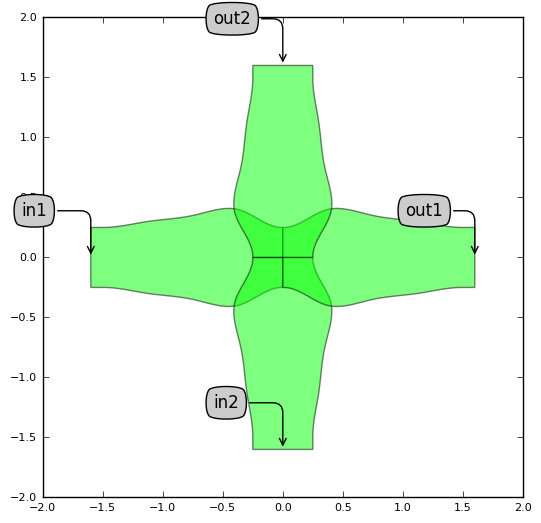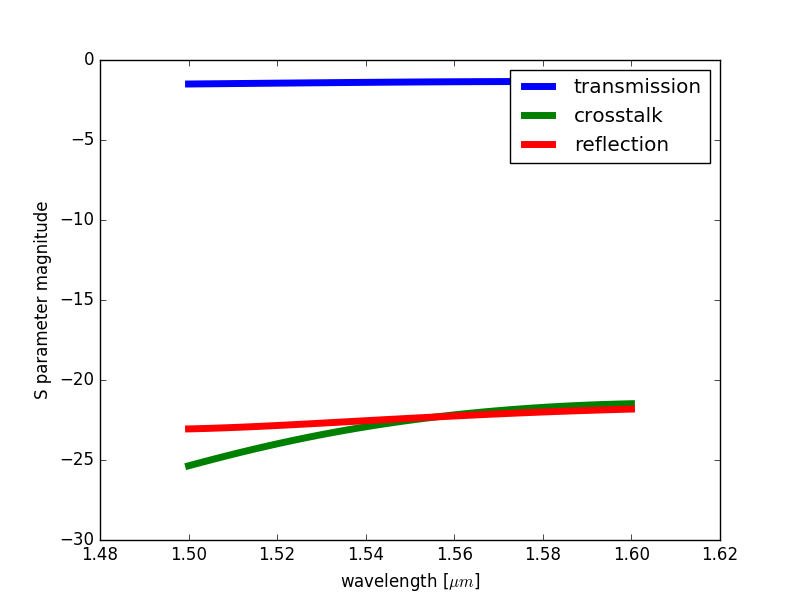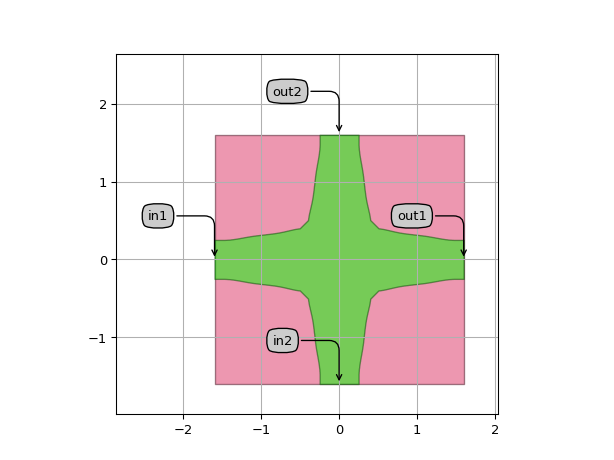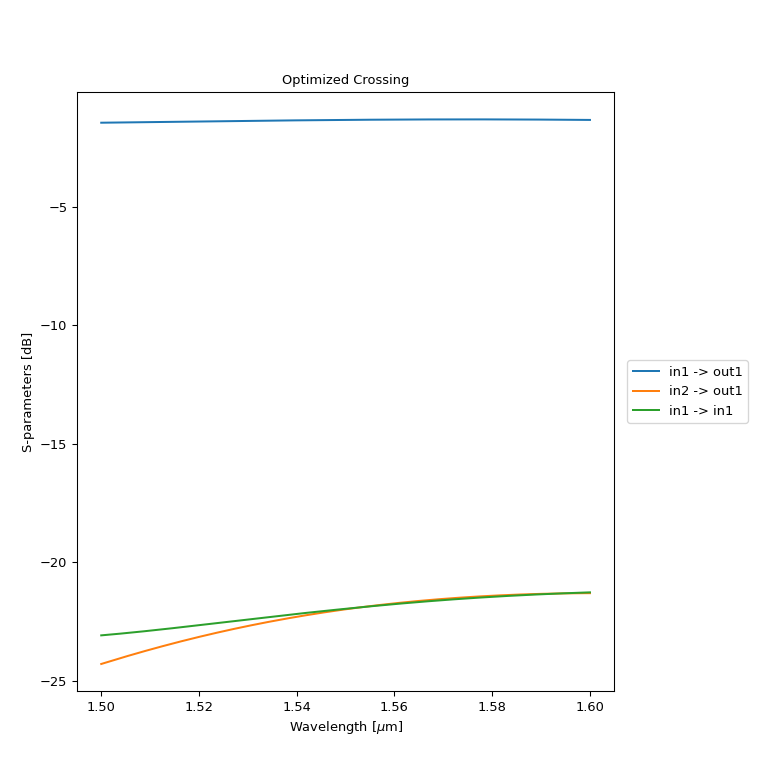# Waveguide crossing¶

SiFab contains two waveguide crossing components:

• Crossing: a parametric waveguide crossing.
• CrossingOptimized: an optimized waveguide crossing with fixed properties, that has been pre-simulated using Ansys Lumerical FDTD.

## Crossing¶

This crossing is completely parametric and builds up from splines with equidistant waypoints, separated by an amount given by the parameter segment_length. The height of the way-points is determined by the parameter widths. The crossing is calculated to be completely symmetric along the X and the Y axes. This parametric crossing has no circuit model.

Reference

Click on the name of the component below to see the complete PCell reference.

 si_fab.all.Crossing Low-loss waveguide crossing.

Example

from si_fab import all as pdk

# Parametric Crossing

crossing = pdk.Crossing(name="Crossing",
widths=[0.5, 0.8, 0.7, 0.8, 0.5],
segment_length=0.375,
min_straight=0.1,
discretisation=0.01)

crossing_lv = crossing.Layout()
crossing_lv.visualize(annotate=True)### Simulation¶

Stand-alone simulations of the Crossing PCell can be performed by applying the simulation recipe of the crossing on a parametric layout. Usually, this is done to explore the component behaviour when the parameters are changed, or to optimize it. As simulations can take time to complete, it is best to organise and save all the simulation results in one folder for later inspection. This allows to run long simulations once and process the data later.

Reference

Click on the name of the function below to see the complete PCell reference.

 si_fab.components.crossing.simulation.simulate_lumerical.simulate_crossing Simulates a crossing with Lumerical.and returns a smatrix.

Example

Listing 37 si_fab/components/crossing/doc/example_crossing_simulation.py
import si_fab.all as pdk
from si_fab.components.crossing.simulation.simulate_lumerical import simulate_crossing
import joblib
import os
import pylab as plt
import numpy as np

inspect = False  # Inspect the simulation
resimulate = True  # Resimulate
project_folder = "./crossing_sim"  # Name of the project
smatrix_path = os.path.join(project_folder, "smatrix.z")  # Path where the smatrix is saved

if not os.path.exists(project_folder):
os.mkdir(project_folder)

cell = pdk.Crossing(name="Crossing")
lv = cell.Layout()
fig = lv.visualize(annotate=True, show=False)
fig.savefig(os.path.join(project_folder, "crossing_sim_layout.png"),
transparent=True,
bbox_inches='tight')

if resimulate:
smatrix = simulate_crossing(layout=lv, project_folder=project_folder, inspect=inspect)
joblib.dump(smatrix, smatrix_path)

if inspect is False:
wavelengths = smatrix.sweep_parameter_values

def dB(x): return 20 * np.log10(np.abs(x))
plt.figure()
plt.plot(wavelengths, dB(smatrix["out1", "in1"]), label='transmission', linewidth=5)
plt.plot(wavelengths, dB(smatrix["out1", "in2"]), label='crosstalk', linewidth=5)
plt.plot(wavelengths, dB(smatrix["in1", "in1"]), label='reflection', linewidth=5)
plt.legend()
plt.xlabel(r'wavelength [$\mu m$]')
plt.ylabel('S parameter magnitude')
plt.savefig(os.path.join(project_folder, "model.png"), transparent=True)## CrossingOptimized¶

CrossingOptimized is a pre-simulated component with locked properties. The simulated S-matrix is used by the circuit model to run Caphe simulations.

Reference

Click on the name of the component below to see the complete PCell reference.

 si_fab.all.CrossingOptimized Optimized low-loss waveguide crossing.

Example

from si_fab import all as pdk
import pylab as plt
import numpy as np

def dB(x): return 20 * np.log10(np.abs(x))

# Optimized Crossing

crossing = pdk.CrossingOptimized()

# Layout

crossing_lv = crossing.Layout()
crossing_lv.write_gdsii("crossing.gds")
crossing_lv.visualize(annotate=True)

# Circuit simulation

crossing_cm = crossing.CircuitModel()

wavelengths_sim = np.linspace(1.50, 1.6, 1000)
smat = crossing_cm.get_smatrix(wavelengths=wavelengths_sim)
plt.figure()
plt.plot(wavelengths_sim, dB(smat["out1", "in1"]), label='transmission', linewidth=5)
plt.plot(wavelengths_sim, dB(smat["out1", "in2"]), label='crosstalk', linewidth=5)
plt.plot(wavelengths_sim, dB(smat["in1", "in1"]), label='reflection', linewidth=5)
plt.legend()
plt.title("Optimized Crossing")
plt.xlabel(r'wavelength [$\mu m$]')
plt.ylabel('S parameter magnitude')
plt.show()